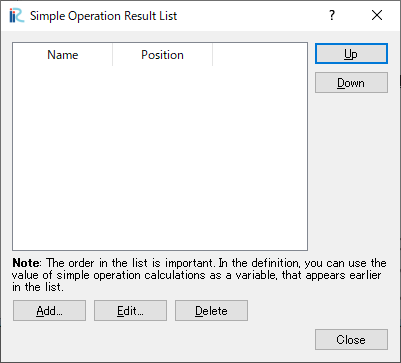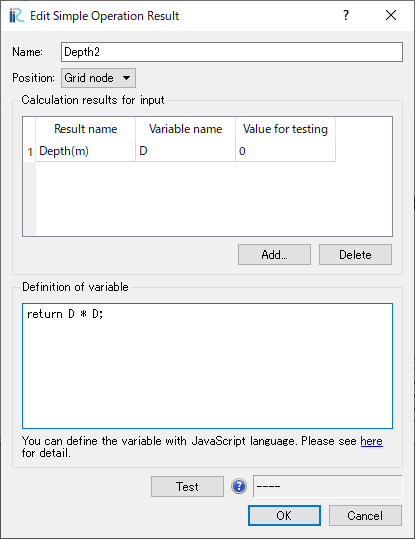# [Calculation Result] (R)¶

The functions of the items under the [Calculation Results] menu are explained in the following sections.

## [Open New 2D Post-processing Window]¶

Description: Opens a new [2D Post-processing Window].

Refer to 2D visualization functions for operations related to [2D Post-processing Window].

## [Open New Bird’s Eye 2D Post-processing Window]¶

Description: Opens a new [Bird’s Eye 2D Post-processing Window].

Refer to Bird’s-Eye View 2D Visualization Functions for operations related to [Bird’s Eye 2D Post-processing Window].

## [Open New 3D Post-processing Window]¶

Description: Opens a new 3D Post-processing Window.

Refer to 3D visualization functions for operations related to [3D Post-processing Window].

## [Open New Graph Window]¶

Description: Opens a new [Graph Window].

Refer to [Graph Window] for operations related to [Graph Window].

## [Open New Scattered Chart Window]¶

Description: Opens a new [Scattered Chart Window].

Refer to [Scattered Chart Window] for operations related to [Scattered Chart Window].

## [Compare with measured values]¶

Description: Opens a dialog to compare measured values with calculation result.

Refer to [Compare with measured values] for operations related to [Compare with measured values].

Description: Reload calculation result.

When you select [Reload], the calculation result is reloaded, and if new calculation results are output by the solver, the timesteps on [Animation Toolbar] is updated.

## [Delete] (D)¶

Description: Delete calculation result.

When you select [Delete], a dialog to confirm whether you really want to delete the calculation results opens. Click on [Yes] to delete calculation result.

## [Manage simple operation results] (M)¶

Description: Manage simple operation results.

Simple operation results are values defined as results of simple numerical operations between calculation results.

“Simple Operation Result List” dialog ( Figure 100) is shown, and you can manage simple operation result list on this dialog.Figure 100 “Simple Operation Result List” dialog

### Adding and editing simple operation results¶

On “Simple Operation Result List” dialog, you can open “Edit Simple Operation Result” dialog (Figure 101) by clicking on “Add” or “Edit” button.

Name: Please input the name of the simple operation result

Calculation results for input: You can add or delete calculation results for input by clicking on “Add” or “Delete” button below. Please refer to Table 12 for detail on the items in the table.

Definition of variable: Please describe how to calculate the simple operation result value, by JavaScript language. Please refer to Examples of definition of simple operation result for examples of how to describe the definition. You can use the variables defined in “Calculation results for input” as input of the definition.

When you click on “Test” button, the value of simple operation result is calculated from the content of “Definition of variable” and the values of “Value for testing” in “Calculation results for input”. If the definition contains problems, an error message is shown.Figure 101 “Edit Simple Operation Result” dialog

Table 12 Detail of “Calculation results for input”
Item name Description
Result name The name of calculation result for input. You can select from combobox
Variable name The name of variable that you can use to refer the value in “Definition of variable”
Value for testing The value that is input into the variable when the “Test” button is clicked

### Examples of definition of simple operation result¶

Examples of simple operation result definitions is shown here.

You can define simple operation results by using functions like below:

• Simple operators (List 3)
• JavaScript built-in functions (List 4)
• Control syntaxes like if, while (List 5)
• User defined functions (List 6)

Please refer to web pages for detail of JavaScript language, like the link below:

https://developer.mozilla.org/en/docs/Web/JavaScript

List 3 Examples of definition of simple operation result (Simple operators)
return D * D;

List 4 Examples of definition of simple operation result (Built-in functions)
return Math.sqrt(D);

List 5 Examples of definition of simple operation result (Control syntaxes)
var d2 = D;
while (d2 < 1000) {
d2 = d2 * 2;
}
if (d2 > 1500) {
d2 = 1500;
}

return d2;

List 6 Examples of definition of simple operation result (User defined functions)
function f1(d) {
return d * d;
}

function f2(d, e) {
if (d < e) {
return e;
} else {
return d;
}
}

return f1(D) * f2(D, E);


## [Import] (I)¶

Description: Imports calculation result.

The function of this item is the same to [Calculation Result] under [Import] menu under [File] menu. Refer to [Calucation Result] (R).

## [Export] (E)¶

Description: Exports the calculation result. Calculation result is exported to VTK files or CSV files.

The function of this item is the same to [Calculation Result] under [Export] menu under [File] menu. Refer to [Calculation Result] (R).

## [Import Visualization/Graph Settings]¶

Description: Imports the settings of visualization windows and graph windows.

The function of this item is the same to [Visualization/Graph Settings] under [Import] menu under [File] menu. Refer to [Visualization/Graph Settings] (V).

## [Export Visualization/Graph Settings]¶

Description: Exports the settings of visualization windows and graph windows.

The function of this item is the same to [Visualization/Graph Settings] under [Export] menu under [File] menu. Refer to [Visualization/Graph Settings].